library(data.table)
library(tidyverse)
library(scales)
library(gsDesign)
library(kableExtra)

Typically the sample size of a study is fixed, ideally based on a power analysis. We collect all our data then analyse it once with the appropriate statistical model. This is an ideal, which people often fall short of in practice. It’s common for scientists and analysts alike to check in on an experiment as the data is coming in (Albers, 2019, Miller, 2010). There’s a lot of potential for error here if experiments are stopped or changed based on ad hoc peeks at the data. One way to address this issue is to go down the pre-registration route, where the full experimental protocol is specified in advance and published for others to see. This makes it harder to divert from your plan and not have people notice.

Another option is to allow interim analyses as the data accumulates. Sequential analysis describes a range of techniques that allow researchers to carry out interim analyses. An experiment may then be stopped by these interim analyses, meaning the sample size is variable, rather than fixed. The point of sequential analysis techniques is that they allow you to test sequentially in a principled way, rather than randomly peeking at the data (see Albers, 2019 for an introduction to sequential testing).

Sequential analysis is broad topic covering a wide range of techniques (Whitehead, 2005 provides a nice historical overview). This post will focus on group sequential designs as a method for sequential analysis. An outline for these methods is provided below, after introducing the problem sequential analysis addresses.

# The problem with naive early stopping

Previously I outlined a set of functions to simulate A/B tests with a binary outcome. These functions are used here, so if you’re interested in how they work, go read the previous post.

To begin with, let’s simulate a set of experiments. We’ll simulate 1,000 experiments each lasting 4 weeks, with 500 new people each week. The base-rate in the control group is set to 50%, and there is no difference between treatment and control (treatment_effect = 0).

set.seed(37)

df_tests <-
sim_tests(
n_per_week = 500,
baseline = 0.5,
treatment_effect = 0,
n_weeks = 4,
n_tests = 1000
) %>%
as_tibble()

Now there’s a set of tests, some naive early stopping can be added. Here a flag is added to indicate weeks where the $$p$$-value for a prop test is < 0.05, and the experiment would be stopped.

# add early stopping to tests
df_tests_stop <- df_tests %>%
# flag for whether p-value is less than 0.05
mutate(stop = as.numeric(p_value < 0.05)) %>%
group_by(test) %>%
# first p-value stopped at, if stop == 0 for
# all weeks this will be the p-value for the first week
mutate(stop_p_value = first(p_value, desc(stop))) %>%
ungroup()

Overall across all the weeks and tests 5% of $$p$$-values are less than 0.05. However, once we add early stopping into the mix the error rate goes up. In this case, 12% of tests have at least 1 week where $$p$$ < 0.05. By running 4 significance tests on each experiment, the chances of a false-positive increase. This is just another flavour of the multiple comparison problem. If you make lots of comparisons, but don’t correct for it, your error rates are inflated 1.

## Bonferroni

A simple way to control error rates is Bonferonni correction. Here the $$p$$-values are adjusted by multiplying them by the number of comparisons and keeping the 0.05 significance threshold. Equivalently, we can think of this correction as reducing the significant threshold from 0.05 to $$0.05 / 4 = 0.0125$$. For example, if a $$p$$-value is 0.02 then this is greater than 0.05 when multiplied by 4, or greater than the 0.0125 adjusted threshold.

df_tests_stop_bonferroni <- df_tests_stop %>%
mutate(
p_value_bonferroni = pmin(p_value * 4, 1),
stop_bonferroni = as.numeric(p_value_bonferroni < 0.05)
) %>%
group_by(test) %>%
mutate(stop_p_value_bonferonni = first(p_value_bonferroni, desc(stop_bonferroni))) %>%
ungroup()

Using this as the stopping criteria leads to a false positive rate of 3%, which is back where we’d want it. Here we can note that the false-positive rate with Bonferroni correction is less than 5%. As with multiple comparisons, Bonferroni correction in sequential analysis is overly conservative (Anderson et al., 2011, gsDesign Manual).

# Group sequential designs

One set of methods for sequential analysis are group sequential designs. Such designs involve “considering each interim analysis as a miniature fixed sample analysis” (Whitehead, 2005, p.2) where the false-positive rate (called the ‘nominal $$p$$-value’) is set at some level determined by the overall sequential design. Clearly, the significance level for the interim analysis cannot just be set to the standard 0.05, as this will result in false positive rates > 5% due to multiple comparisons. Instead lower significant thresholds needs to be used for the interim analyses. There are a range of methods for defining the critical values for interim analyses.

The three methods described below all proceed from a similar place.

1. Define K, the number of sequential analyses you plan to do.
2. At each interim analysis calculate a Z-statistic for the difference between treatment and control.
3. Compare the Z-statistic to some critical value for that analysis.
4. If the statistic exceeds the critical value, stop the experiment. Otherwise keep collecting data until an interim analysis is significant, or the final analysis is complete.

How the methods differ, is the definition of the critical values for the interim analyses, and, consequently, the sample size required for the sequential design.

The package gsDesign among a number of others (see CRAN Task View: Clinical Trial Design, Monitoring, and Analysis), offers tools for group sequential designs. gsDesign implements a wide range of sequential testing techniques beyond those discussed here, which we’ll look into in a future post. The extensive gsDesign manual can be found wherever you install the package in the doc directory (gsDesign/doc/gsDesignManual.pdf) 2.

## Pocock boundaries

The Pocock method uses the same critical value for all interim analyses. The method is also symmetrical, in that we have the same upper and lower boundary. Other techniques, not covered here, allow you to have different boundaries for the treatment being worse (futility) than the treatment being better (superiority). It’s also possible to use the Pocock method for a one-sided test, where we’re only interested in changes in one direction.

We can easily get the critical values for this design using the gsDesign::gsDesign(). By setting k = 4 we’re saying that there will be four interim analyses, including the last one. test.type = 2 means that we want boundaries for a two-sided symmetrical test. The plot below shows the critical values for the upper and lower boundaries 3. Note that the critical value of 2.36 is higher than the typical 1.96 for a single Z-test. It is also lower than the critical value for Bonferroni correction, which would be qnorm(1 - 0.025 / 4) = 2.498 for the upper boundary.

design_pocock <- gsDesign(k = 4,
test.type = 2,
sfu = 'Pocock')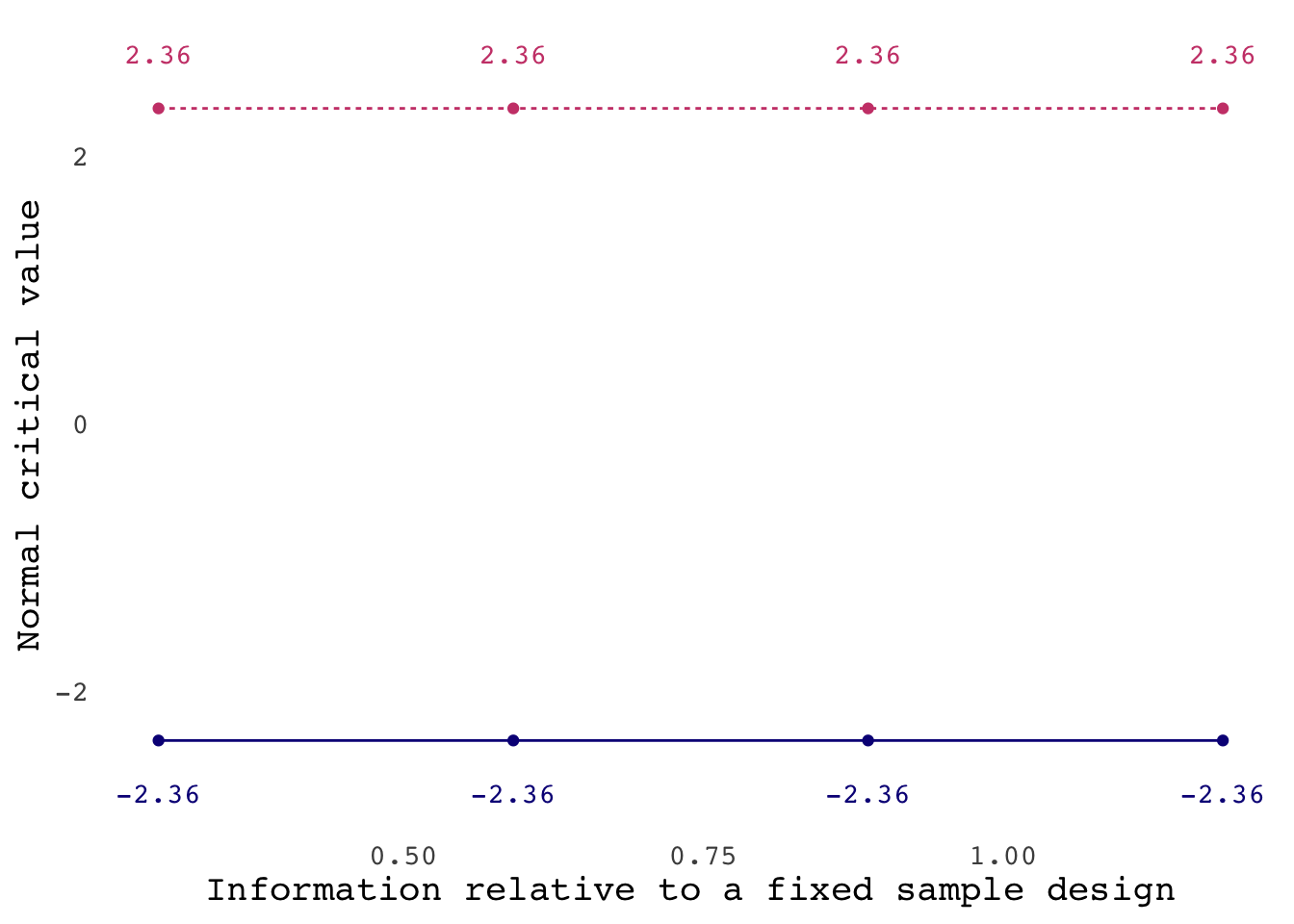If we doubled the number of analyses, then the critical value for each analysis will increase accordingly.

design_pocock_8 <- gsDesign(k = 8,
test.type = 2,
sfu = 'Pocock')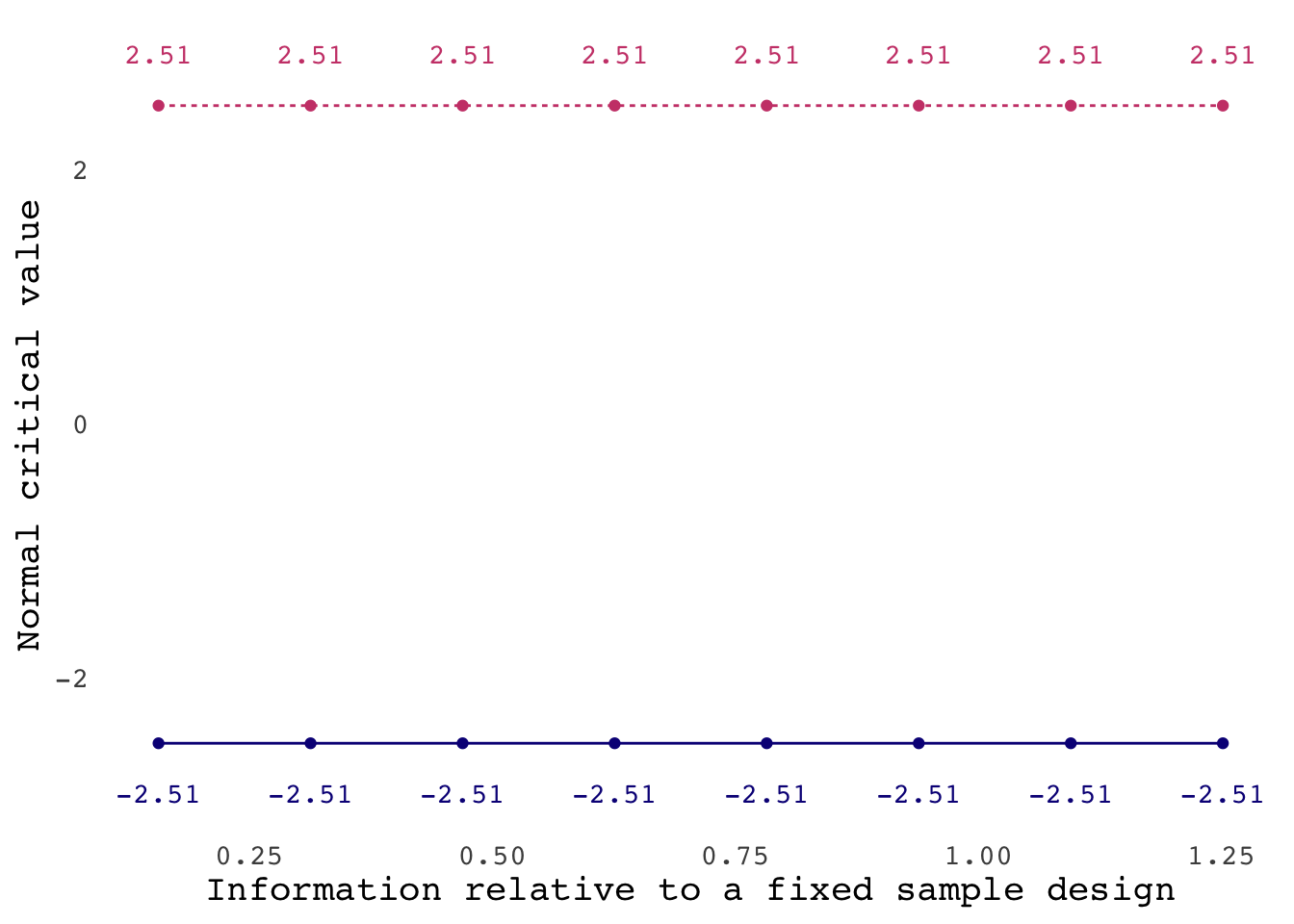gsDesign also provides us with the tools to calculate sample sizes. By printing out the design object we can see that there’s a sample size ratio column and that the note tells us:

“Sample size ratio compared to fixed design with no interim”

design_pocock
## Symmetric two-sided group sequential design with
## 90 % power and 2.5 % Type I Error.
## Spending computations assume trial stops
## if a bound is crossed.
##
##            Sample
##             Size
##   Analysis Ratio*  Z   Nominal p  Spend
##          1  0.296 2.36    0.0091 0.0091
##          2  0.592 2.36    0.0091 0.0067
##          3  0.887 2.36    0.0091 0.0051
##          4  1.183 2.36    0.0091 0.0041
##      Total                       0.0250
##
## ++ alpha spending:
##  Pocock boundary.
## * Sample size ratio compared to fixed design with no interim
##
## Boundary crossing probabilities and expected sample size
## assume any cross stops the trial
##
## Upper boundary (power or Type I Error)
##           Analysis
##    Theta      1      2      3      4 Total   E{N}
##   0.0000 0.0091 0.0067 0.0051 0.0041 0.025 1.1561
##   3.2415 0.2748 0.3059 0.2056 0.1136 0.900 0.6975
##
## Lower boundary (futility or Type II Error)
##           Analysis
##    Theta      1      2      3      4 Total
##   0.0000 0.0091 0.0067 0.0051 0.0041 0.025
##   3.2415 0.0000 0.0000 0.0000 0.0000 0.000

What this mean is that we need to calculate the sample size required to achieve the desired power for our test, then be prepared to collect 1.183x this sample size, if we make it to the final analysis. Using nNormal() we can calculate the sample size required for a simple Z-test where we’re interested in detecting a minimum difference of 0.2 SD with 90% power and a 5% false-positive rate.

n_fixed <- nNormal(
delta1 = 0.2, # 0.2 SD difference
ratio = 1, # 50-50 treatment to control
sided = 2,
sd = 1, # assume the data is standardised
alpha = 0.05, # alpha
beta = 0.1 # 1 - power
) %>%
ceiling()

The plot shows the sample size for a fixed design with the dotted line and the sample required for each interim analysis with the solid line. The first interim analysis is completed when 29.6% of the sample size for a fixed design has been collected. In this case the total sample size required for a fixed design (combining across both groups) would be 1051, meaning the first analysis would be completed at a sample size of 311. If this analysis didn’t cross the boundary then the next analysis would be done when there are 622 cases in total.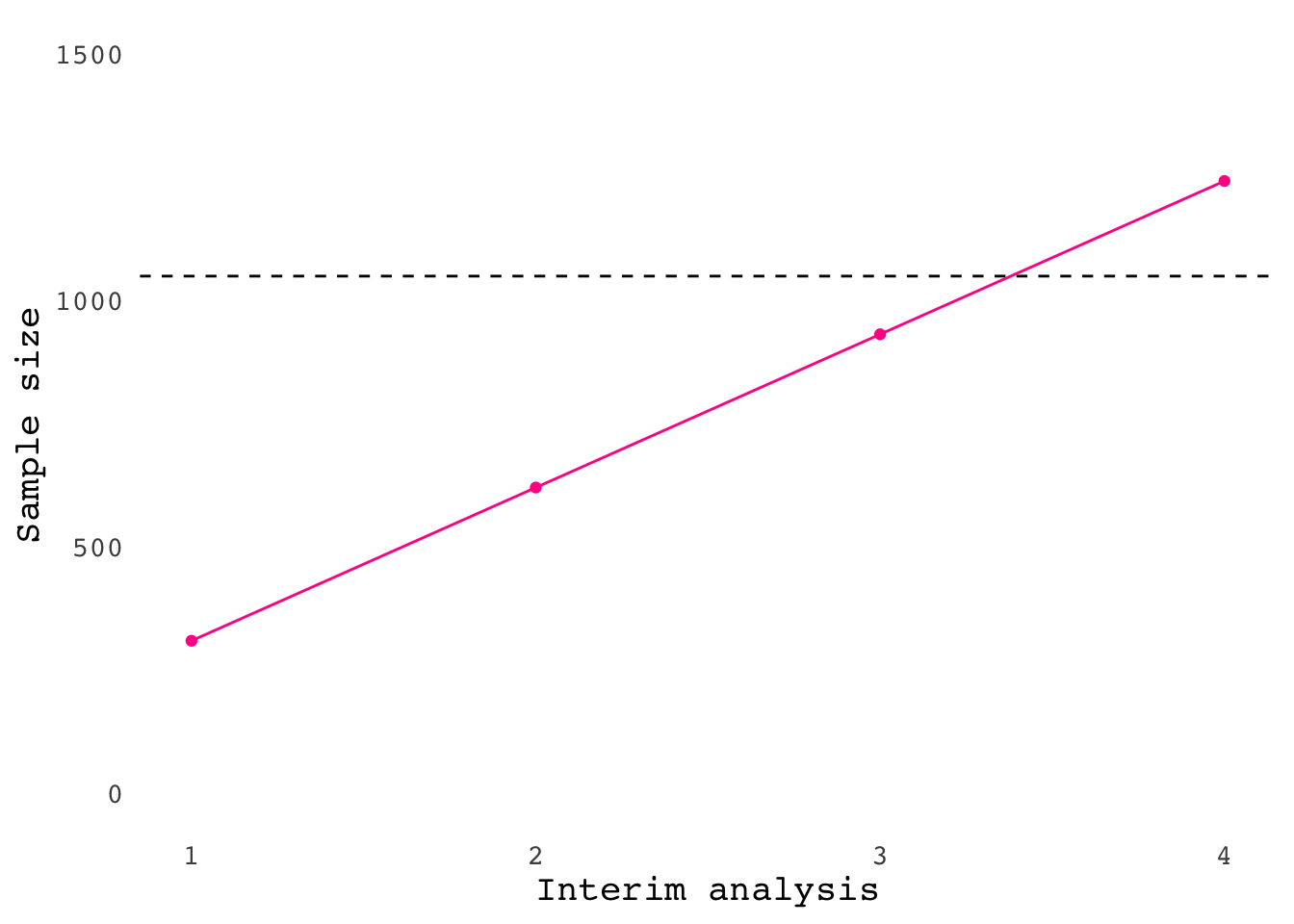## O’Brien-Fleming boundaries

The O’Brien-Fleming method addresses the fact that we are probably reluctant to stop very early in a trial without very strong evidence. Instead of having uniform critical values for all interim analyses, this method has larger critical values for earlier in the experiment. The plot below shows the critical values using the Obrien-Fleming method for four interim analyses. Like the Pocock method, this is a symmetrical design.

design_of <- gsDesign(k = 4,
test.type = 2,
sfu = 'OF')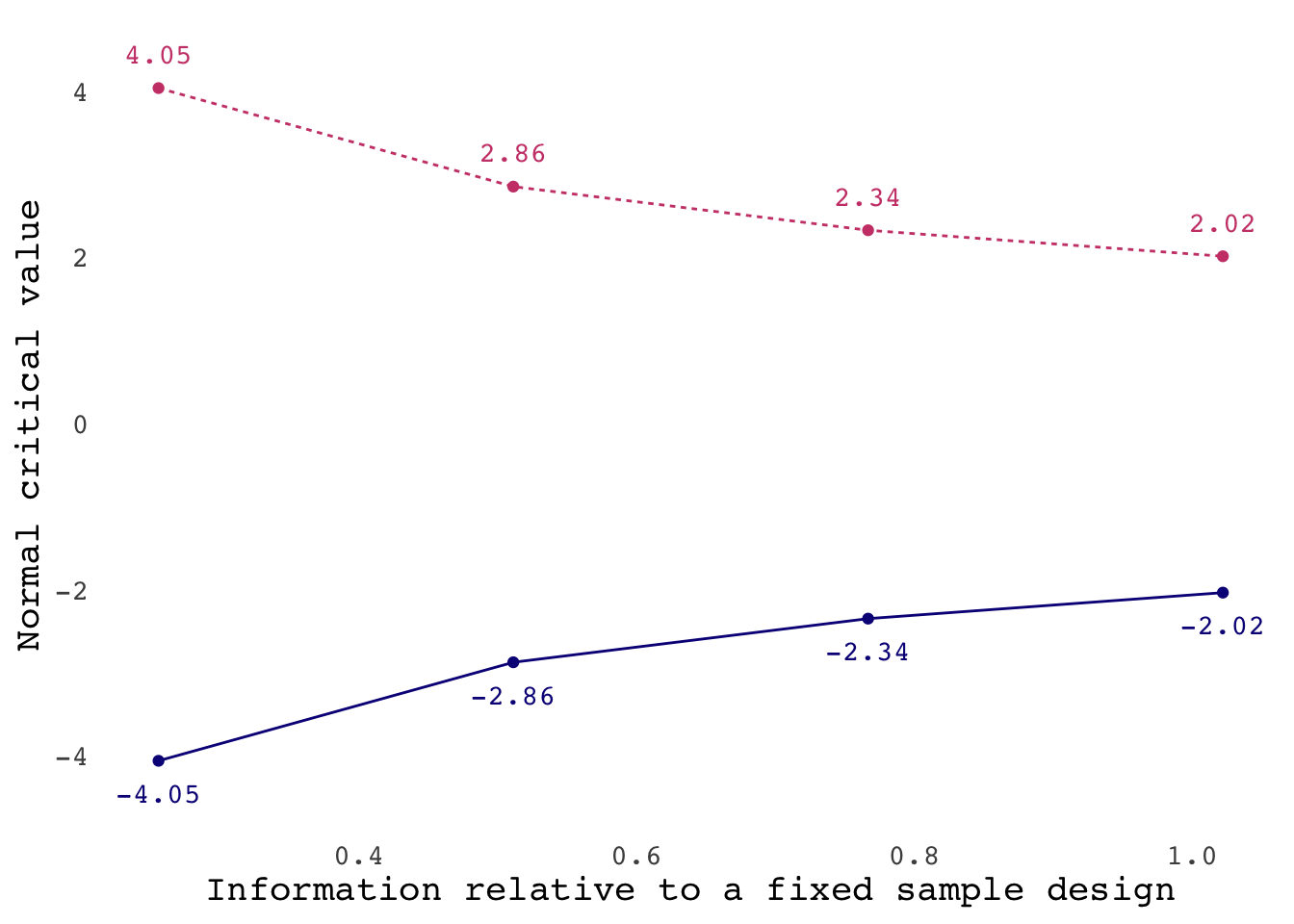Increasing the number of analyses to 8 we can see that the critical value is huge for first analysis.

design_of_8 <- gsDesign(k = 8,
test.type = 2,
sfu = 'OF')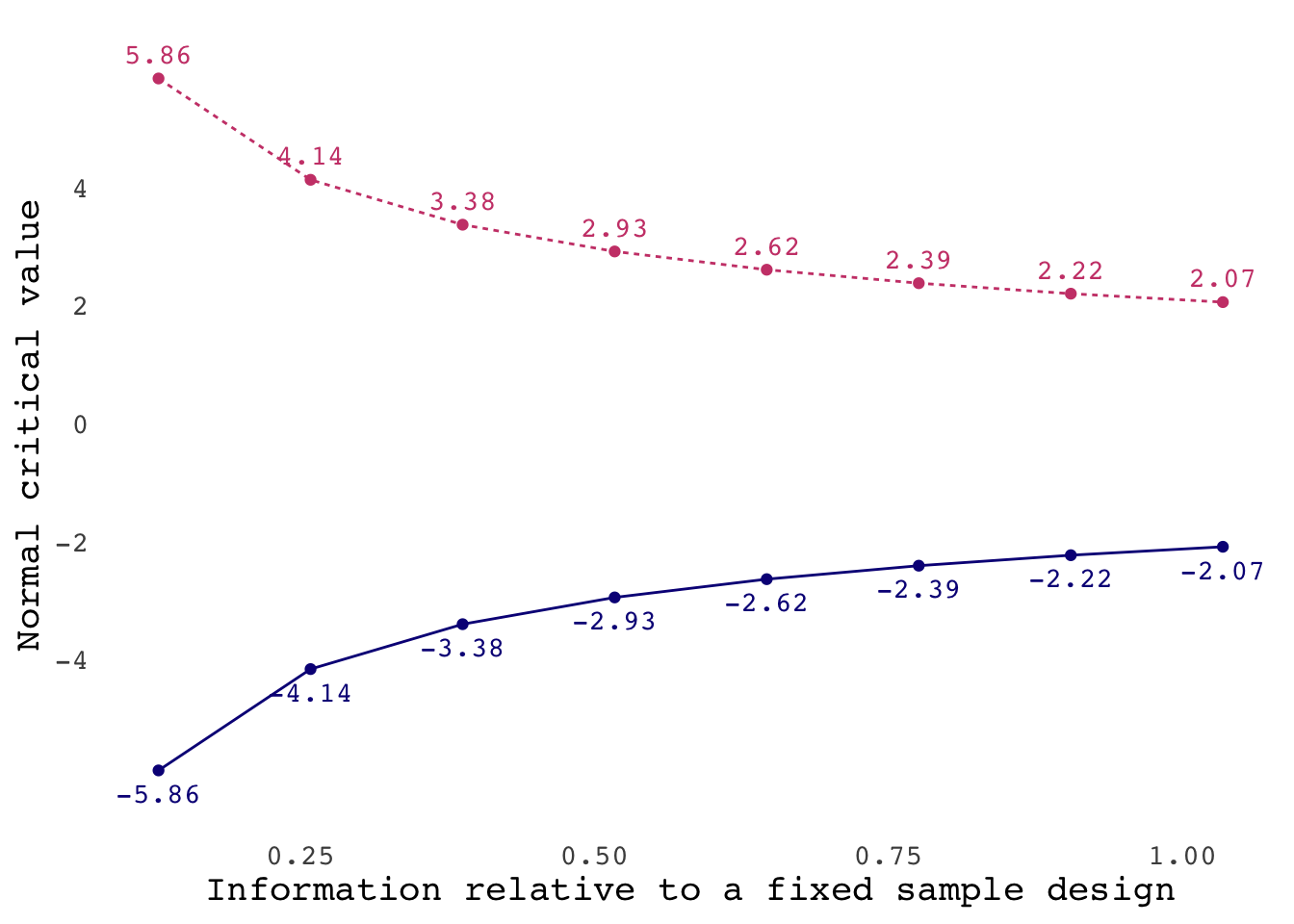Using this method, the required sample size for the final analysis is not much higher than that for a fixed sample design.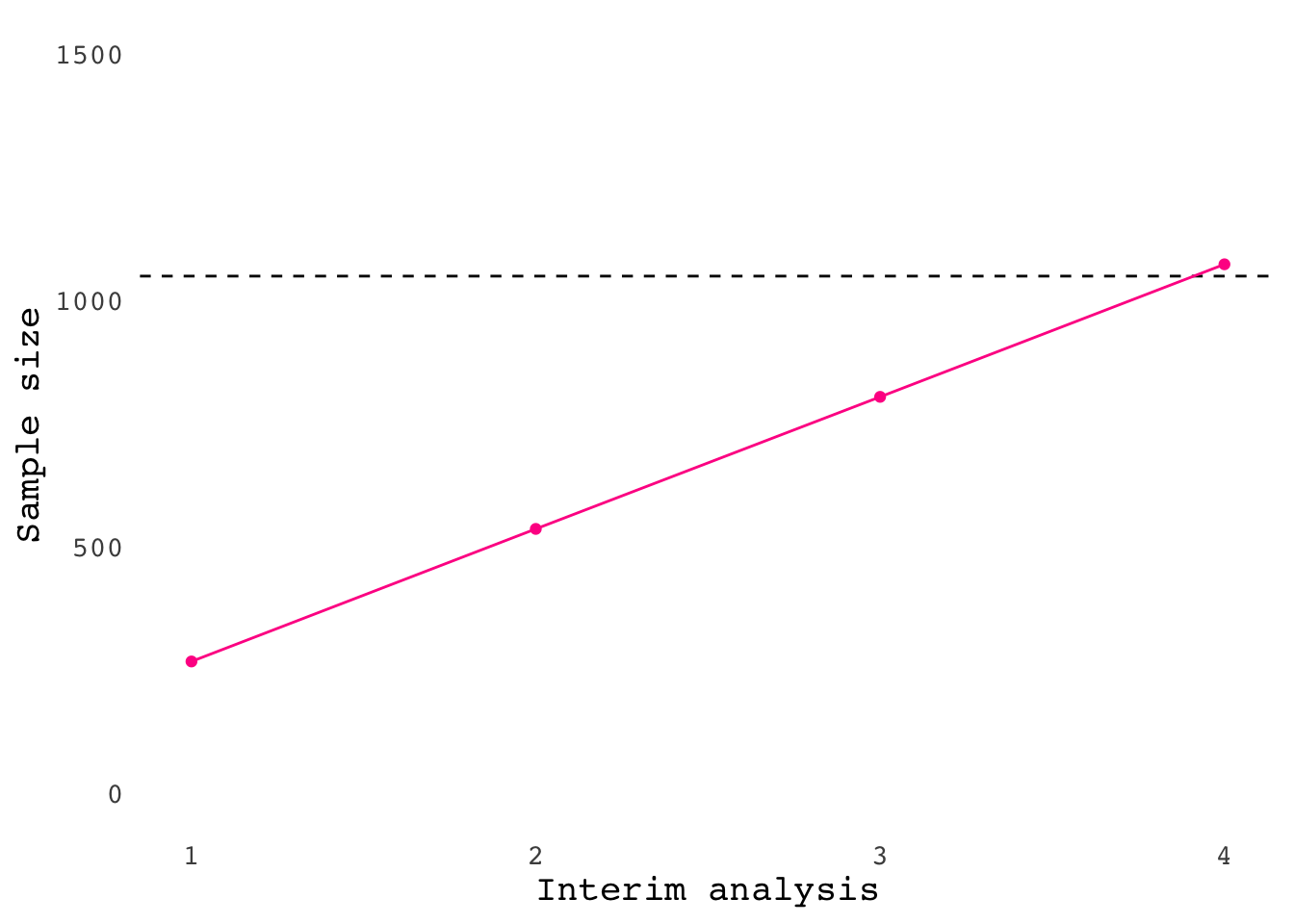## Wang-Tsiatis boundaries

Yet another method with variable critical values is provided by Wang and Tsiatis. Wang and Tsiatis defined a family of boundary functions, where we can control the shape of the boundaries with an additional parameter, delta. When delta is set to 0 we get a O’Brien-Fleming design, and when set to 0.5 we get a Pocock design. Setting to 0.25, as below, gives us something between the two.

design_wt <- gsDesign(
k = 4,
test.type = 2,
sfu = 'WT',
sfupar = 0.25
)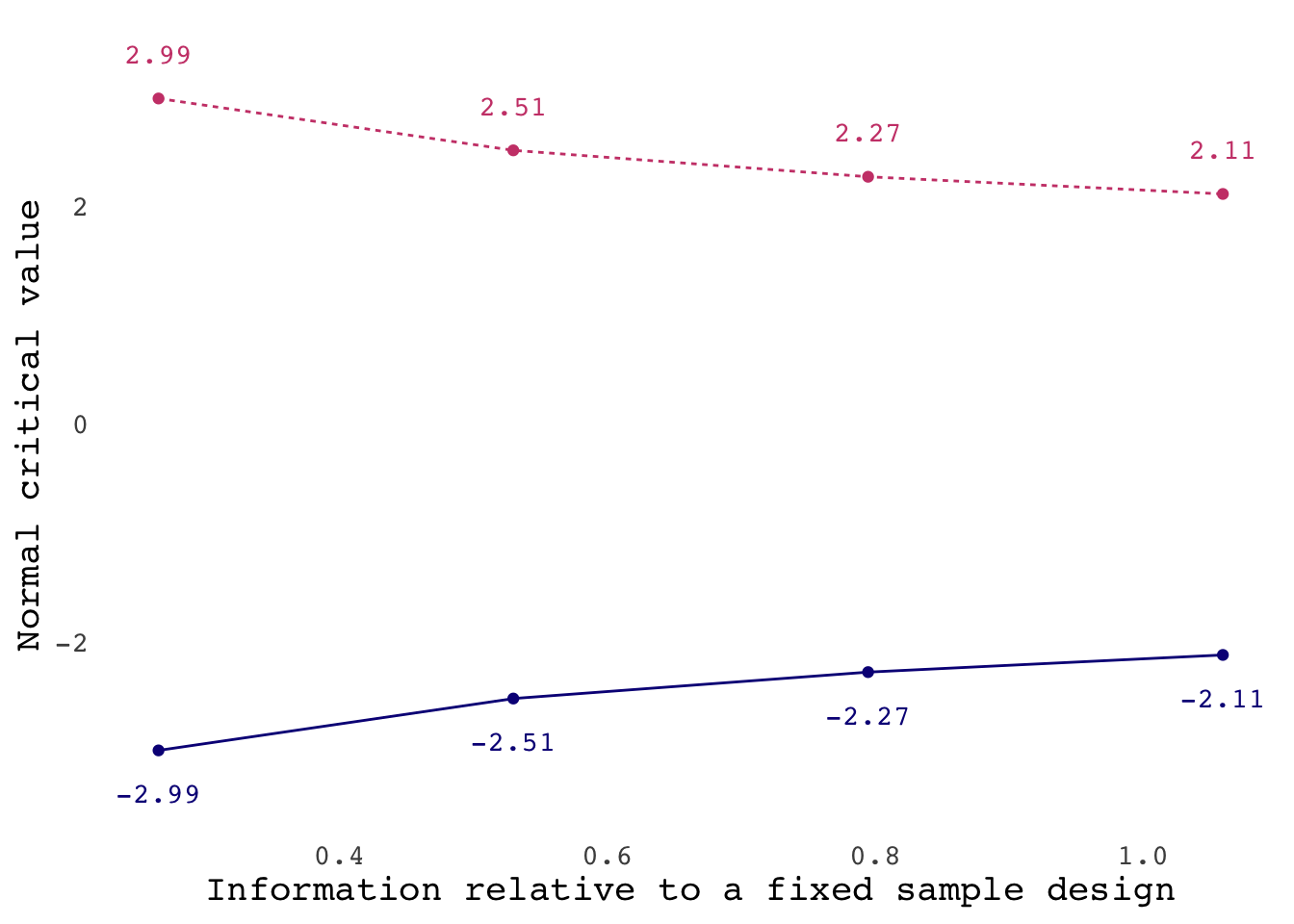The plot below shows the boundaries for changing delta. If you increase delta above 0.5 you end up with lower thresholds for earlier analyses, which doesn’t feel very sensible.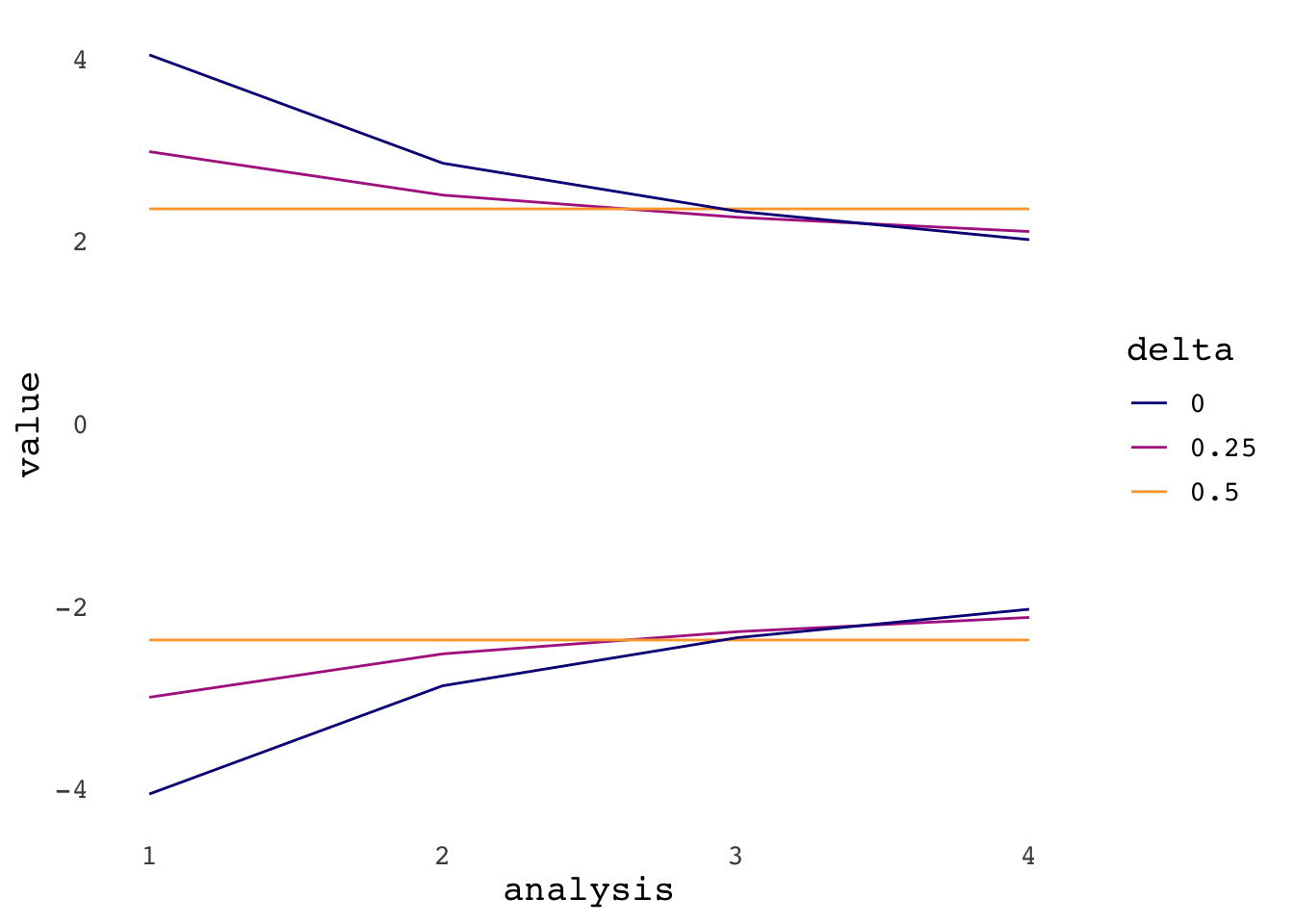The sample size required increases with delta as the boundaries become flatter.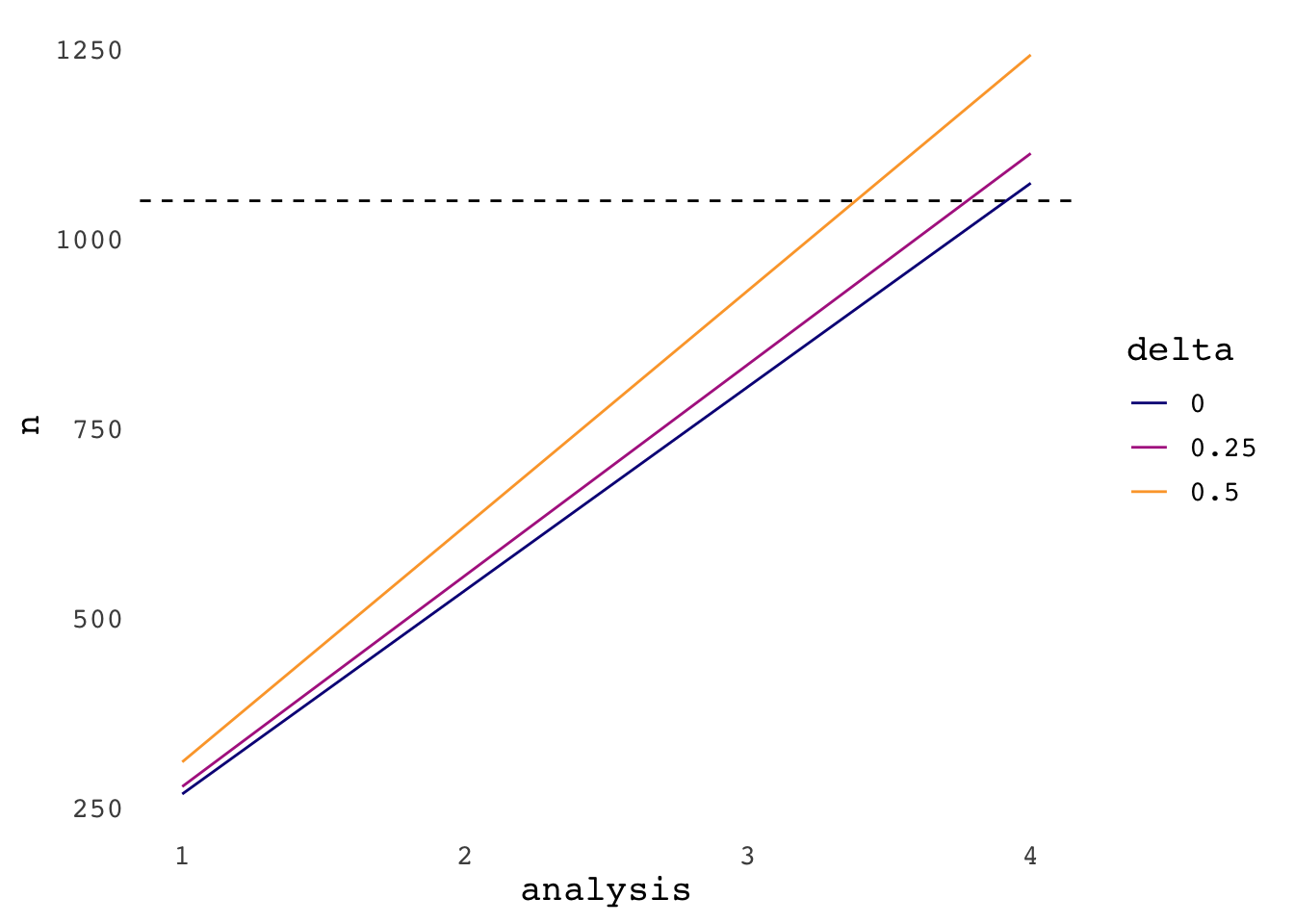# Conclusion

This post has introduced sequential analysis using group sequential designs. A couple of different methods have been explored before finishing with the generalised approach proposed by Wang and Tsiatis. On the one extreme we can use the same threshold for every analysis, as in the early work by Pocock. Alternately we can set more stringent thresholds for earlier analyses, as proposed by O’Brien and Fleming. Wang and Tsiatis boundaries provide a way to vary how stringent the thresholds are for earlier analyses. All these methods are symmetrical with the same boundaries for concluding the treatment is better or worse than control. There are other methods available in gsDesign that do not require this. In addition, some methods do not require you to predefine the sample size at which the interim analyses will be performed. The documentation for gsDesign() offers an introduction to these methods (see Whitehead (2005) and the gsDesign manual for more details) 4.

# Appendix: Normal approximations

This appendix gathers together some thoughts/investigation on using Z-tests for normal approximations.

It may have occurred to you that so far all the critical values and power calculations are for Z-tests. In other words, we aren’t using the more typical t-test to compare continuous outcomes.

As the plot below shows, normal approximations are reasonable for a t-test when samples are large. The pink solid line shows the critical value for a t-distribution for a two-sided test with a 5% false positive rate. The dashed line shows the critical value for a Z-test.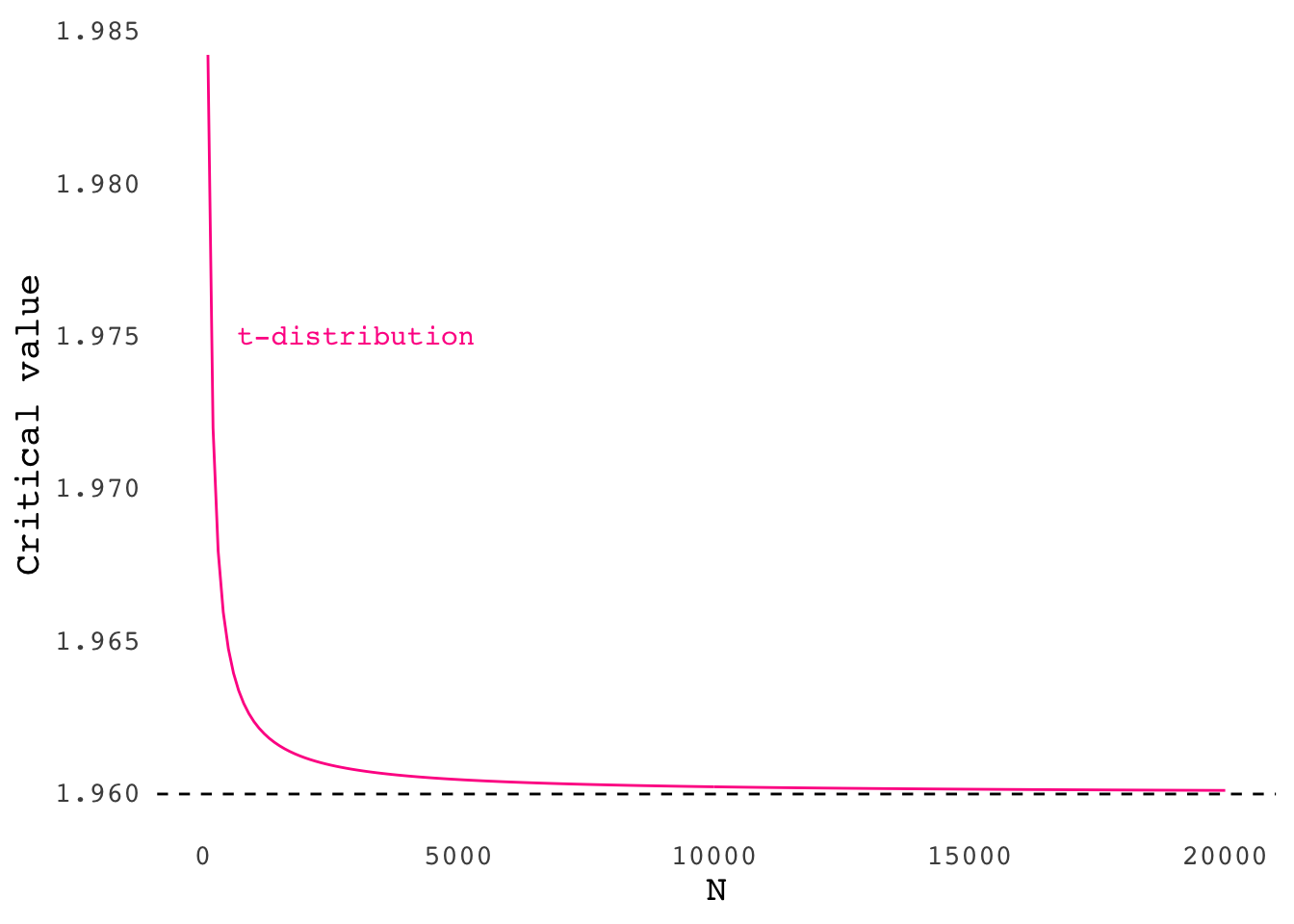Ultimately it’s probably unlikely to have a study that is both sufficiently powered and where using a Z- or t-test makes a substantial difference to the results. Nevertheless, for small samples we could feed the nominal $$p$$-values into qt() to get critical values for a t-distribution. Using an O’Brien-Fleming design, the critical value for the first analysis with N = 100 is then 4.23 instead of 4.05 for a Z-test.

# calculate the nominal p-values
pnorm(design_of$upper$bound, lower.tail = F) %>%
# quantiles for a t-distribution, i.e. critical values
# the absolute version of these values are the upper bounds
qt(df = 99) 
##  -4.233521 -2.930717 -2.376211 -2.050686

gsDesign also provides testBinomial() to calculate a Z-statistic for two proportions. This does something very similar to the more familiar prop.test(). What prop.test() does is calculate a Chi-squared statistic with Yates’ continuity correction. Without this correction the squared-root of the statistic provided by prop.test() is equal to the Z-statistic from testBinomial().

# correct = F to turn off continuity correction
prop.test(c(100, 150), c(1000, 1000), correct = F)
##
##  2-sample test for equality of proportions without continuity
##  correction
##
## data:  c(100, 150) out of c(1000, 1000)
## X-squared = 11.429, df = 1, p-value = 0.0007232
## alternative hypothesis: two.sided
## 95 percent confidence interval:
##  -0.07890532 -0.02109468
## sample estimates:
## prop 1 prop 2
##   0.10   0.15

testBinomial() is set up such that a higher proportion of events in the treatment groups results in a negative Z-statistic. This is presumably geared towards clinical trials where you want to see less of some negative outcome. For this reason the higher number of events for the treatment group is specified to the argument for the control group.

testBinomial(150, 100, 1000, 1000) 
##  3.380617

The Z-statistics of 3.38 is then the same as the square-root of the Chi-squared statistics from the prop.test() without correction.

sqrt(11.429)
##  3.38068

## Comparison of prop.test() and testBinomial()

For small samples Yates’ continuity correction will be more influential making prop.test() more conservative as shown in the plot below. The line shows p-values for prop.test() versus testBinomial() for a sample size of 20, with 10 successes in the control group, and the number of successes in the treatment group varied between 1 and 20. There are cases where the p-value for testBinomial() is less than 0.05 whereas prop.test() gives a p-value over 0.05.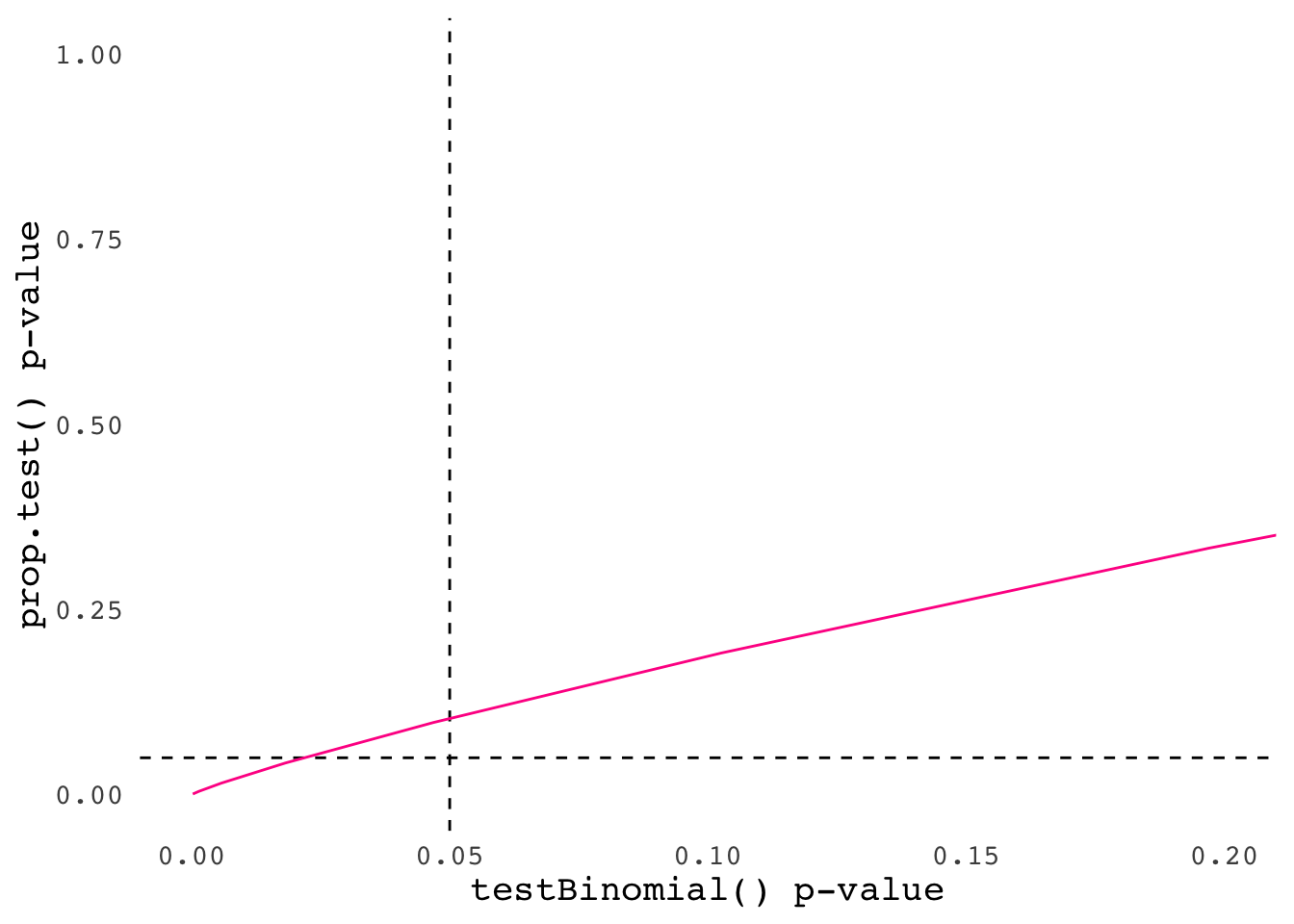For larger samples prop.test() and testBinomial() will give the same result as the continuity correction isn’t having much influence.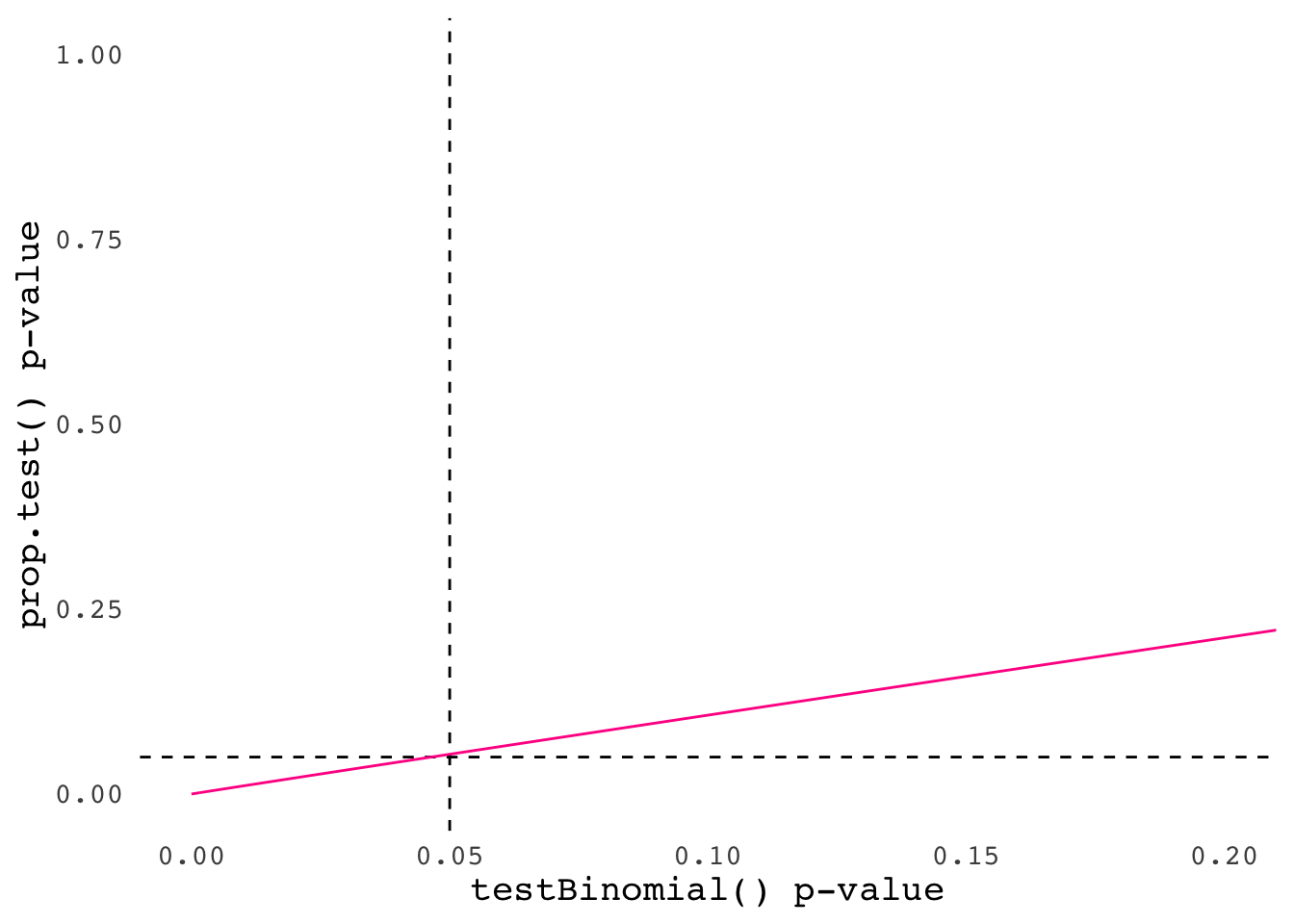1. In cases where there is no difference between groups, 5% of the $$p$$-values will be less than 0.05 in the long-run. This in turn means that 95% of a long-run set of tests will be non-significant at this level. If each test is independent, there’s a $$0.95^4 = 0.81$$ chance that 4 tests are all non-significant. If we take this off one we get a 19% chance that one of four comparisons is significant, which we call the family-wise error rate. This shows why we have to correct for multiple comparisons – the headline error rates only apply to a single test in the long-run. In sequential analyses the tests aren’t independent because the data from subsequent weeks is added on top of the previous week. Without assuming independence, we call still say that the family-wise error rate will be $$FWER \leq 4 \times 0.05 = 0.2$$ (see here).↩︎

2. You can use .libPaths() in R to see where your packages are installed.↩︎

3. I could just use the plot method built into gsDesign here but I wanted to tweak it slightly.↩︎

4. Running gsDesign() %>% plot() will show you an example of an asymmetrical design.↩︎